## Modeling and Simulation at Disparate Scales

### Molten Salt Reactor Multiphysics and International Fuel Cycle Transitions

#### UM NERS Colloquium, 2018.02.16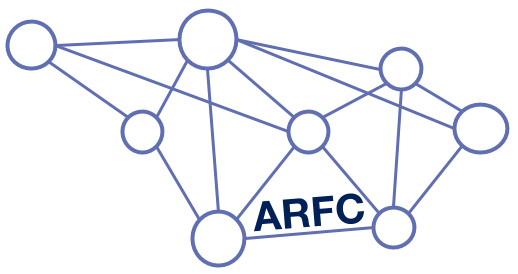### Insights at Disparate Scales### Intro to Moltres/MOOSE

• Fluid-fuelled, molten salt reactors
• Multi-group diffusion (arbitrary groups)
• Advective movement of delayed neutron precursors
• Navier-Stokes thermal hydraulics
• 3D unstructured
• 2D axisymmetric
• 3D structured

### Acquiring Moltres


git clone https://github.com/arfc/moltres
cd moltres
git submodule init
git submodule update


### Acquiring Moltres

Moltres is built atop the Multi-physics Object-Oriented Simulation Environment (MOOSE) (Gaston et al.).

• MOOSE interfaces with libMesh to discretize simulation volume into finite elements
• Provides interface for coding residuals that correspond to weak form of governing PDEs; also interface for coding Jacobians → more accurate Jacobians → more efficient convergence
• Residuals and Jacobians handed off to PetSc which handles solution of resulting non-linear system of algebraic equations

In Moltres, neutrons are described with time-dependent multi-group diffusion:

\begin{align} \frac{1}{v_g}\frac{\partial \phi_g}{\partial t} &- \nabla \cdot D_g \nabla \phi_g + \Sigma_g^r \phi_g = \sum_{g \ne g'}^G \Sigma_{g'\rightarrow g}^s \phi_{g'} + \chi_g^p \sum_{g' = 1}^G (1 - \beta) \nu \Sigma_{g'}^f \phi_{g'} + \chi_g^d \sum_i^I \lambda_i C_i \end{align}
\begin{align} v_g &= \mbox{speed of neutrons in group g} \\ \phi_g &= \mbox{flux of neutrons in group g} \\ t &= \mbox{time} \\ D_g &= \mbox{Diffusion coefficient for neutrons in group g} \\ \Sigma_g^r &= \mbox{macroscopic cross-section for removal of neutrons from group g} \\ \Sigma_{g'\rightarrow g}^s &= \mbox{macroscopic cross-section of scattering from g' to g} \\ \chi_g^p &= \mbox{prompt fission spectrum, neutrons in group g} \\ G &= \mbox{number of discrete groups, g} \\ \nu &= \mbox{number of neutrons produced per fission} \\ \Sigma_g^f &= \mbox{macroscopic cross section for fission due to neutrons in group g} \\ \chi_g^d &= \mbox{delayed fission spectrum, neutrons in group g} \\ I &= \mbox{number of delayed neutron precursor groups} \\ \beta &= \mbox{delayed neutron fraction}\\ \lambda_i &= \mbox{average decay constant of delayed neutron precursors in precursor group i} \\ C_i &= \mbox{concentration of delayed neutron precursors in precursor group i} . \end{align}

Delayed neutron precursors are described to include a term representing the effect of fuel advection.

\begin{align} \frac{\partial C_i}{\partial t} &= \sum_{g'= 1}^G \beta_i \nu \Sigma_{g'}^f \phi_{g'} - \lambda_i C_i - \frac{\partial}{\partial z} u C_i \label{eq:precursors} \end{align}
The governing equation for the fuel temperature is modeled as: \begin{align} \rho_fc_{p,f}\frac{\partial T_f}{\partial t} &+ \nabla\cdot\left(\rho_f c_{p,f} \vec{u}\cdot T_f -k_f\nabla T_f\right) = Q_f \end{align} \begin{align} \rho_f &= \mbox{density of fuel salt}\\ c_{p,f} &= \mbox{specific heat capacity of fuel salt}\\ T_f &= \mbox{temperature of fuel salt}\\ \vec{u} &= \mbox{velocity of fuel salt}\\ k_f &= \mbox{thermal conductivity of fuel salt}\\ Q_f &= \mbox{source term} = \sum_{g=1}^G \epsilon_{f,g}\Sigma_{f,g}\phi_g \end{align}
In the moderator, the governing equation for temperature is given by: \begin{align} \rho_gc_{p,g}\frac{\partial T_g}{\partial t} &+ \nabla\cdot\left(-k_g\nabla T_g\right) = Q_g\\ \end{align} \begin{align} \rho_g &= \mbox{density of graphite moderator}\\ c_{p,g} &= \mbox{specific heat capacity of graphite moderator}\\ T_g &= \mbox{temperature of graphite moderator}\\ k_g &= \mbox{thermal conductivity of graphite moderator}\\ Q_g &= \mbox{source term in graphite moderator}\\ \end{align}

### Moltres (coupling in MOOSE)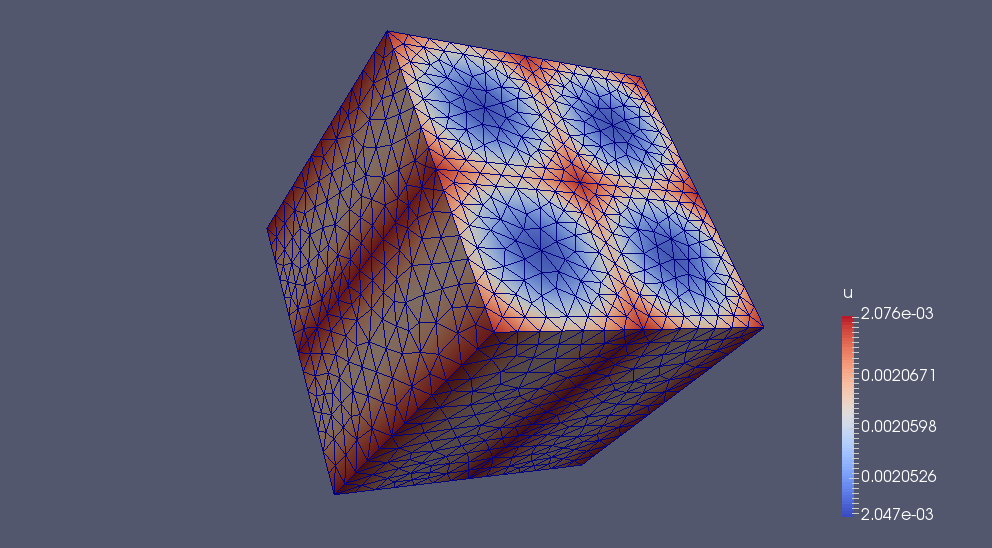Alexander Lindsay, 2016

### Moltres Precursor Drift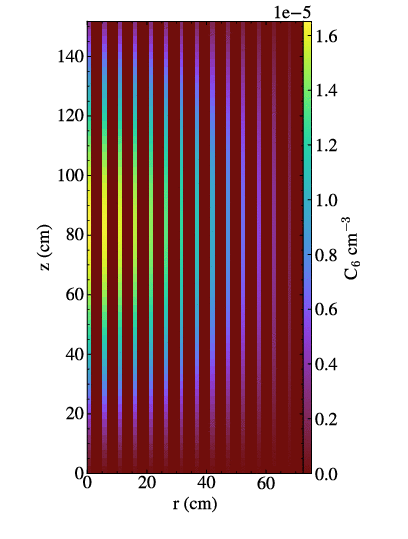### Moltres MSRE Comparison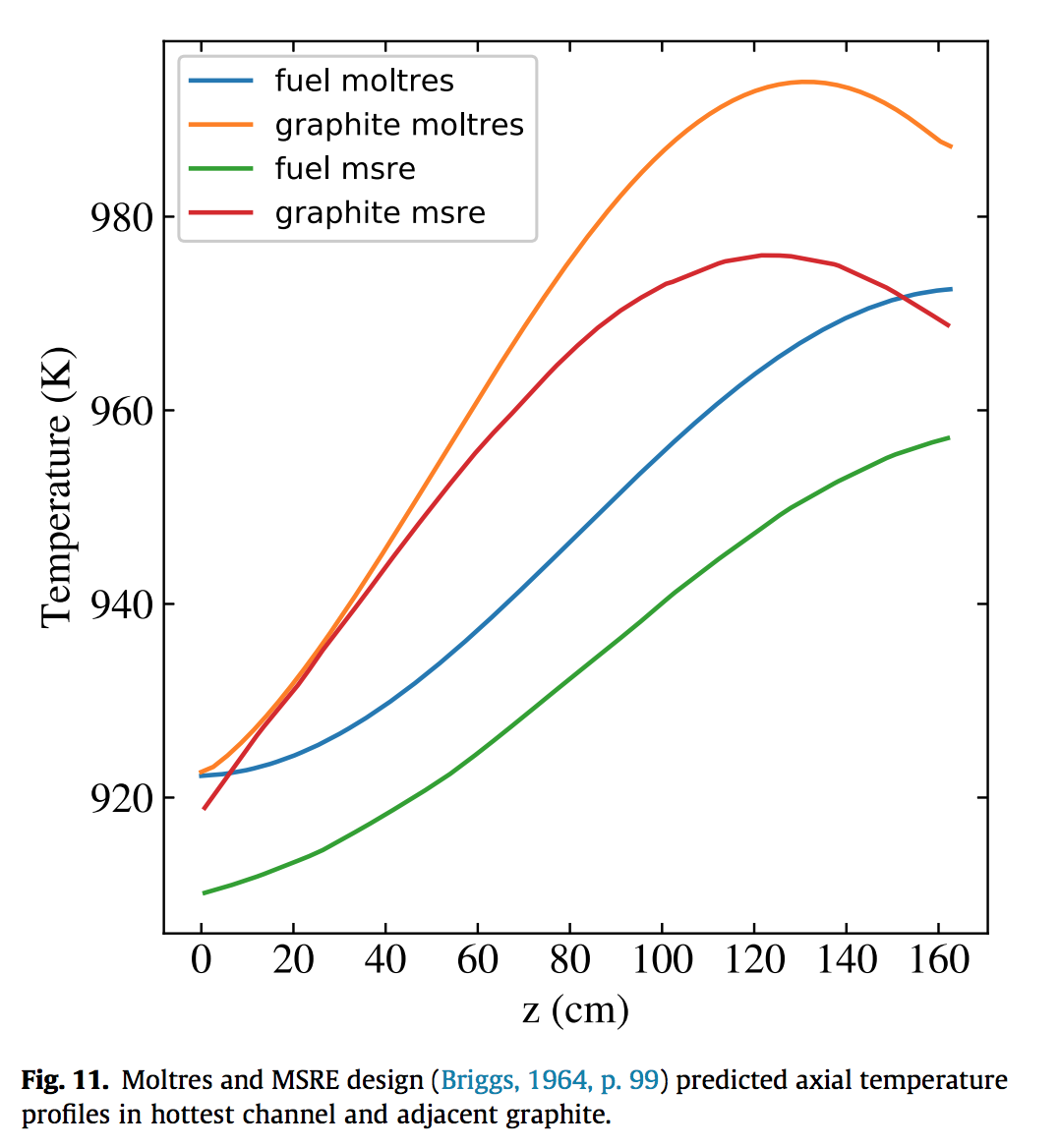### Moltres MSRE Comparison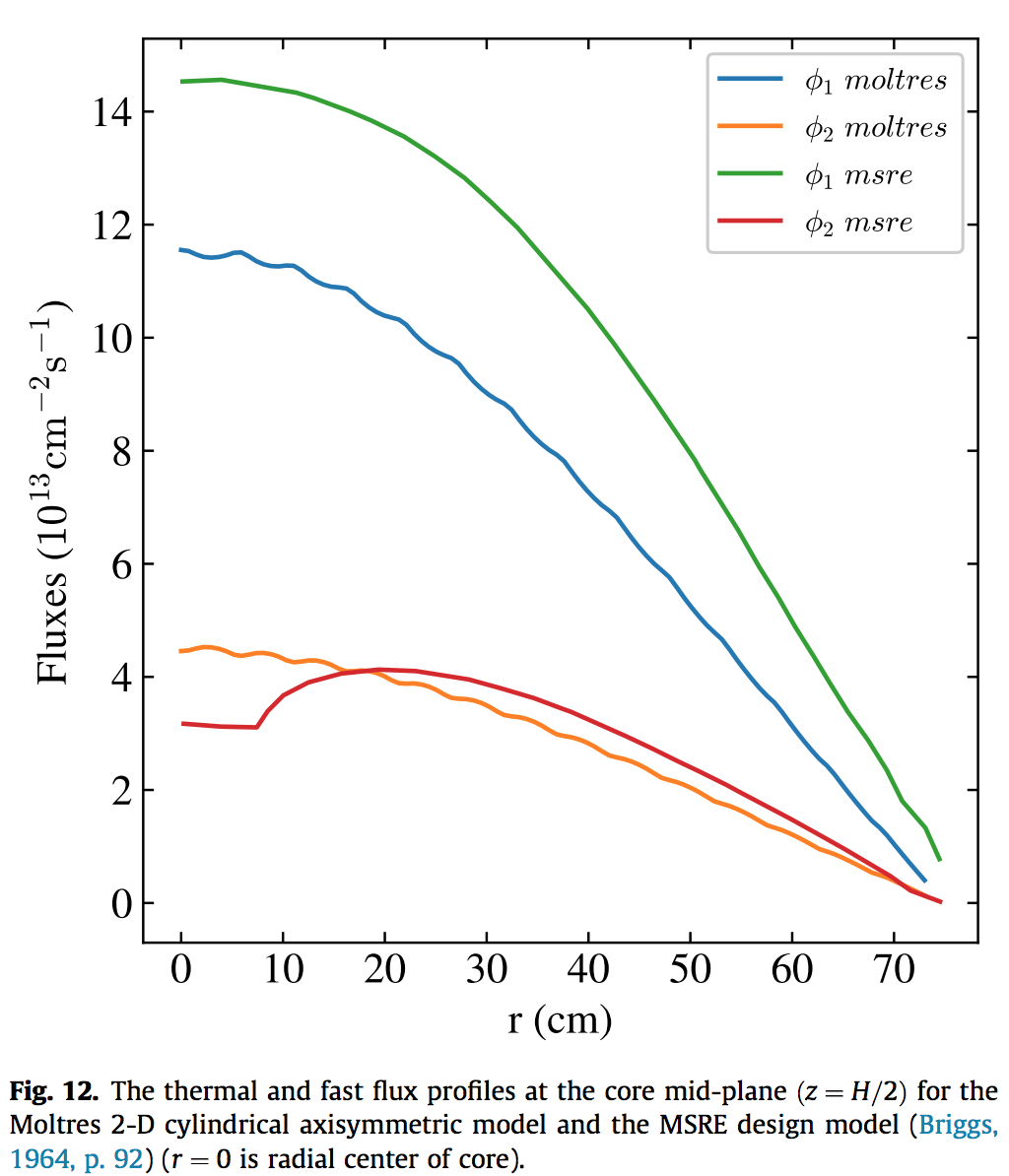### Moltres MSRE Comparison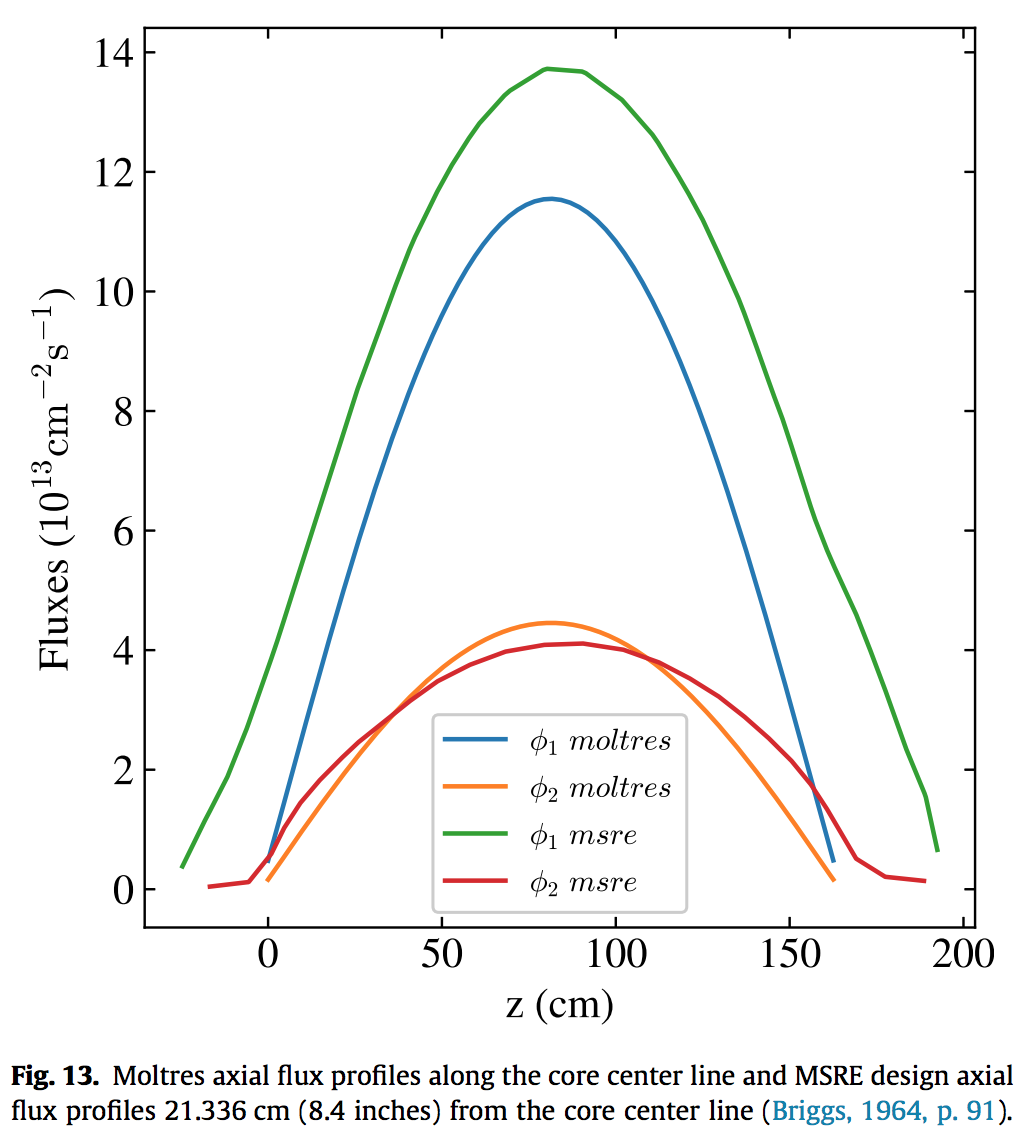### Moltres (cross sections via Serpent)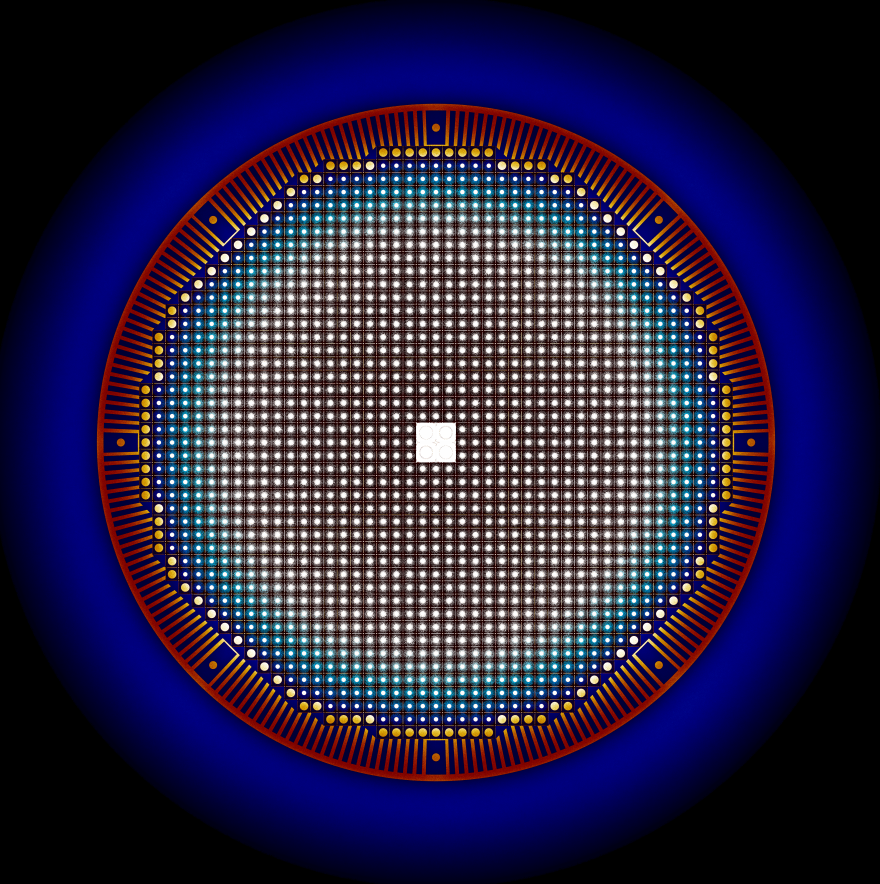Andrei Rykhlevskii, 2017

### Moltres (cross sections via Serpent)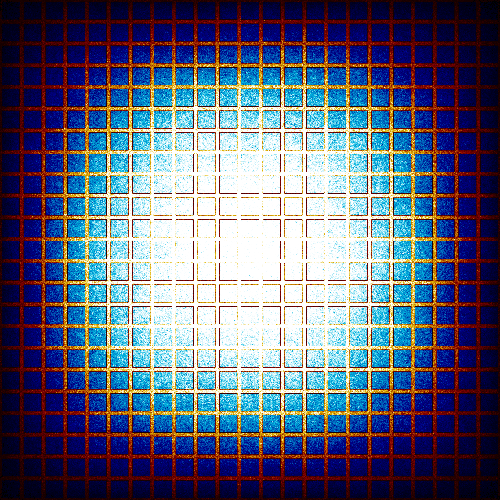Alexander Lindsay, 2016

### Reactor Physics and Serpent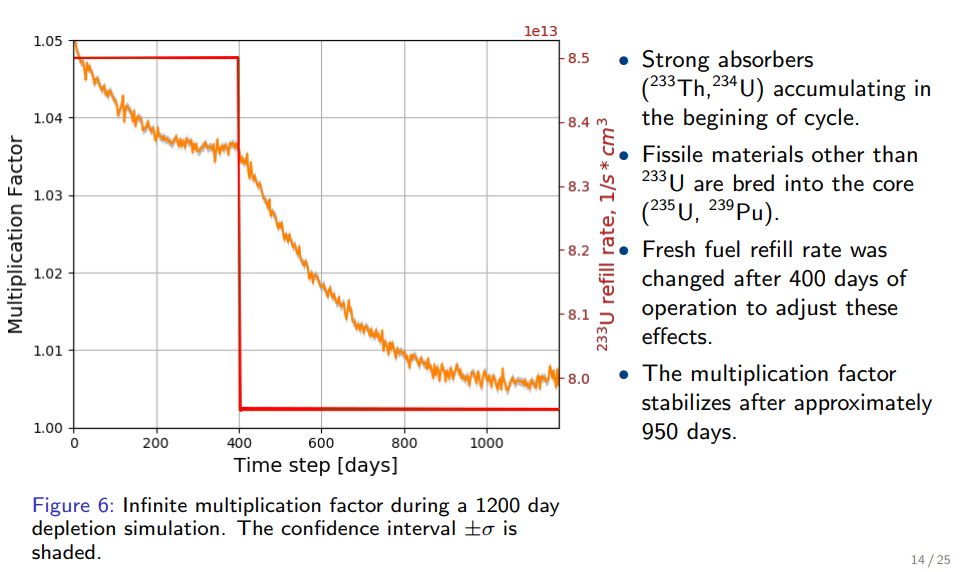Andrei Rykhlevskii, 2017

### Reactor Physics and Serpent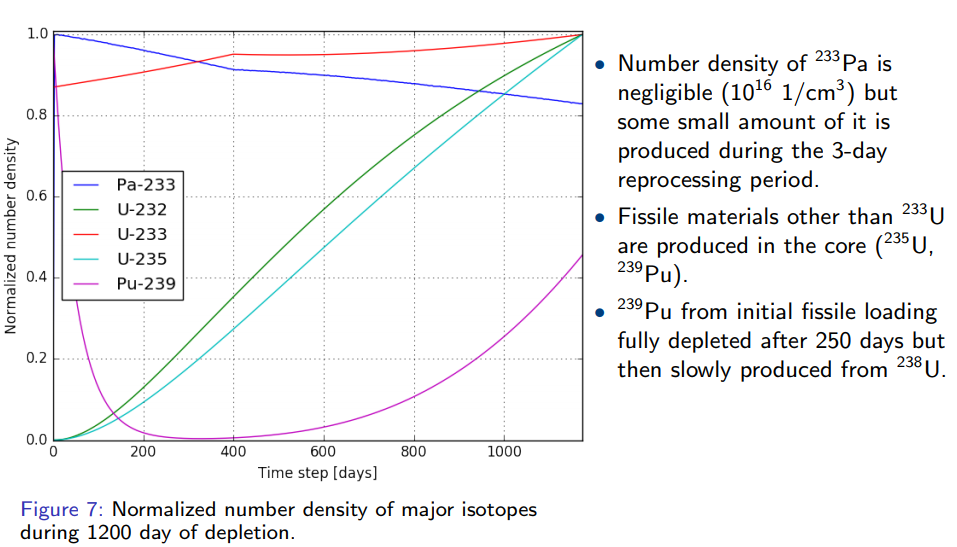Andrei Rykhlevskii, 2017### The Nuclear Fuel Cycle

Hundreds of discrete facilities mine, mill, convert, fabricate, transmute, recycle, and store nuclear material.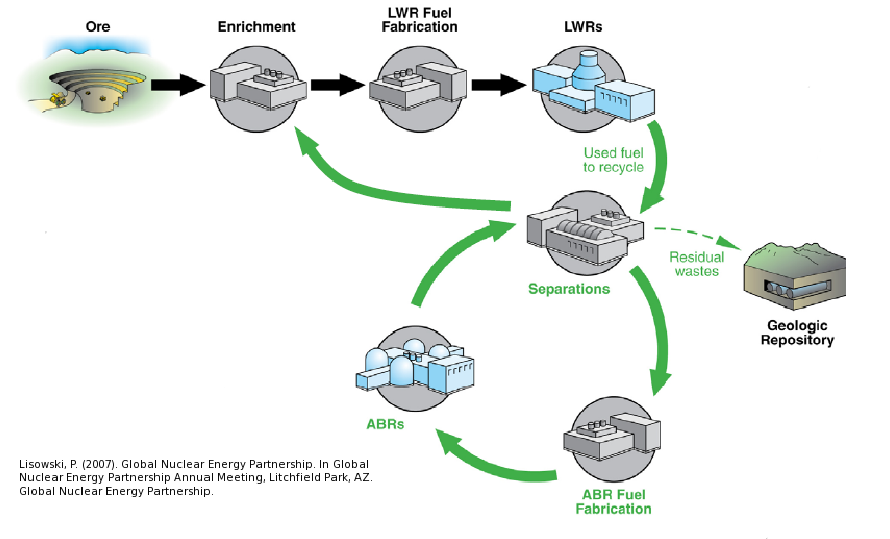### Fuel Cycle Metrics

• Mass Flow
• proliferation resistance and physical protection (PRPP) indices.
• Cost
• levelized cost of electricity,
• facility life cycle costs.
• Economics
• power production, facility deployments,
• dynamic pricing and feedback.
• Disruptions
• reliability, safety,
• system robustness.

### Agent Based Systems Analysis

A facility might create material.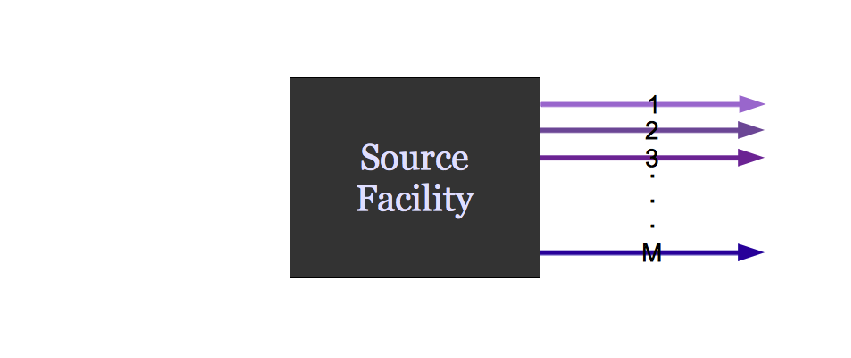### Agent Based Systems Analysis

It might request material.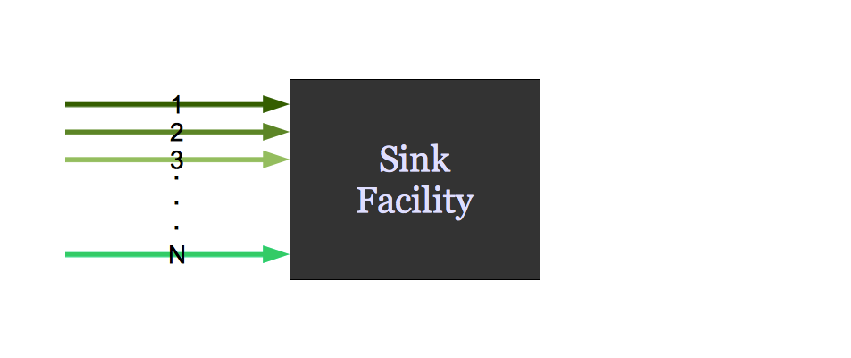### Agent Based Systems Analysis

It might do both.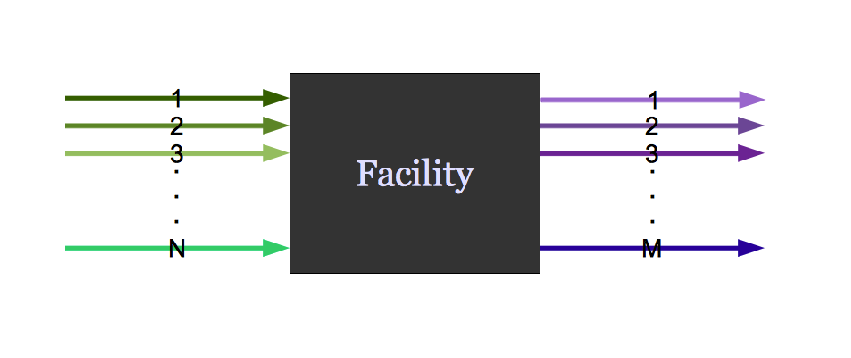### Agent Based Systems Analysis

Even simple fuel cycles have many independent agents.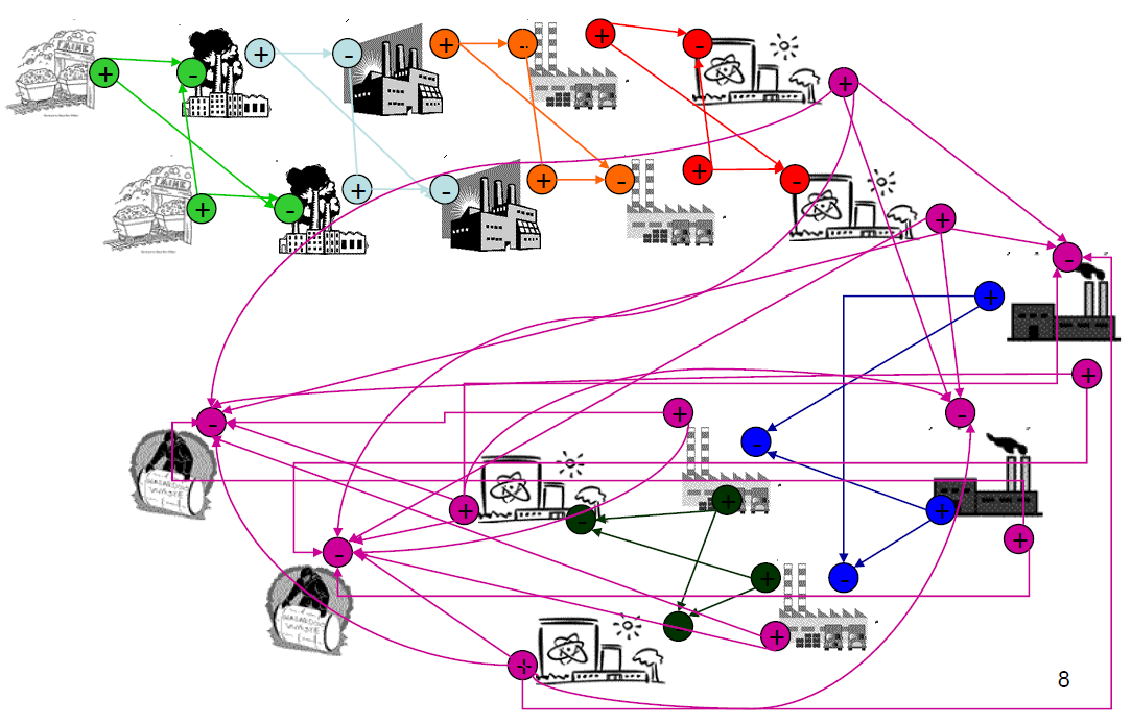### Dynamic Resource Exchange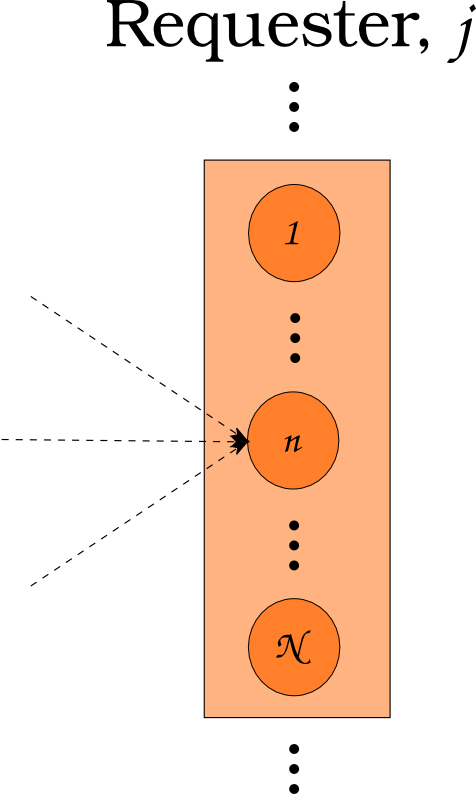$N_i \subset N$

### Dynamic Resource Exchange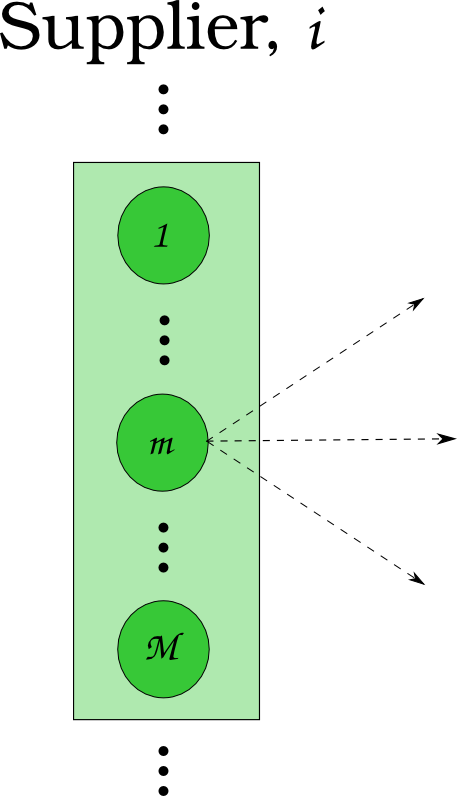$N_j \subset N$

### Dynamic Resource Exchange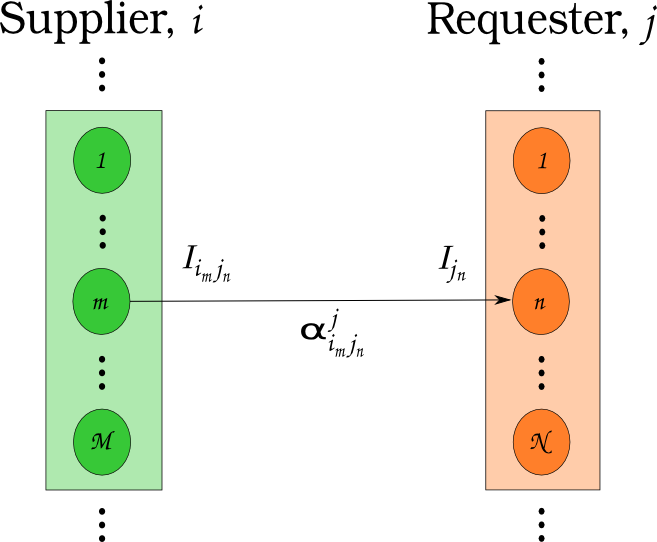$N_i \cup N_j = N$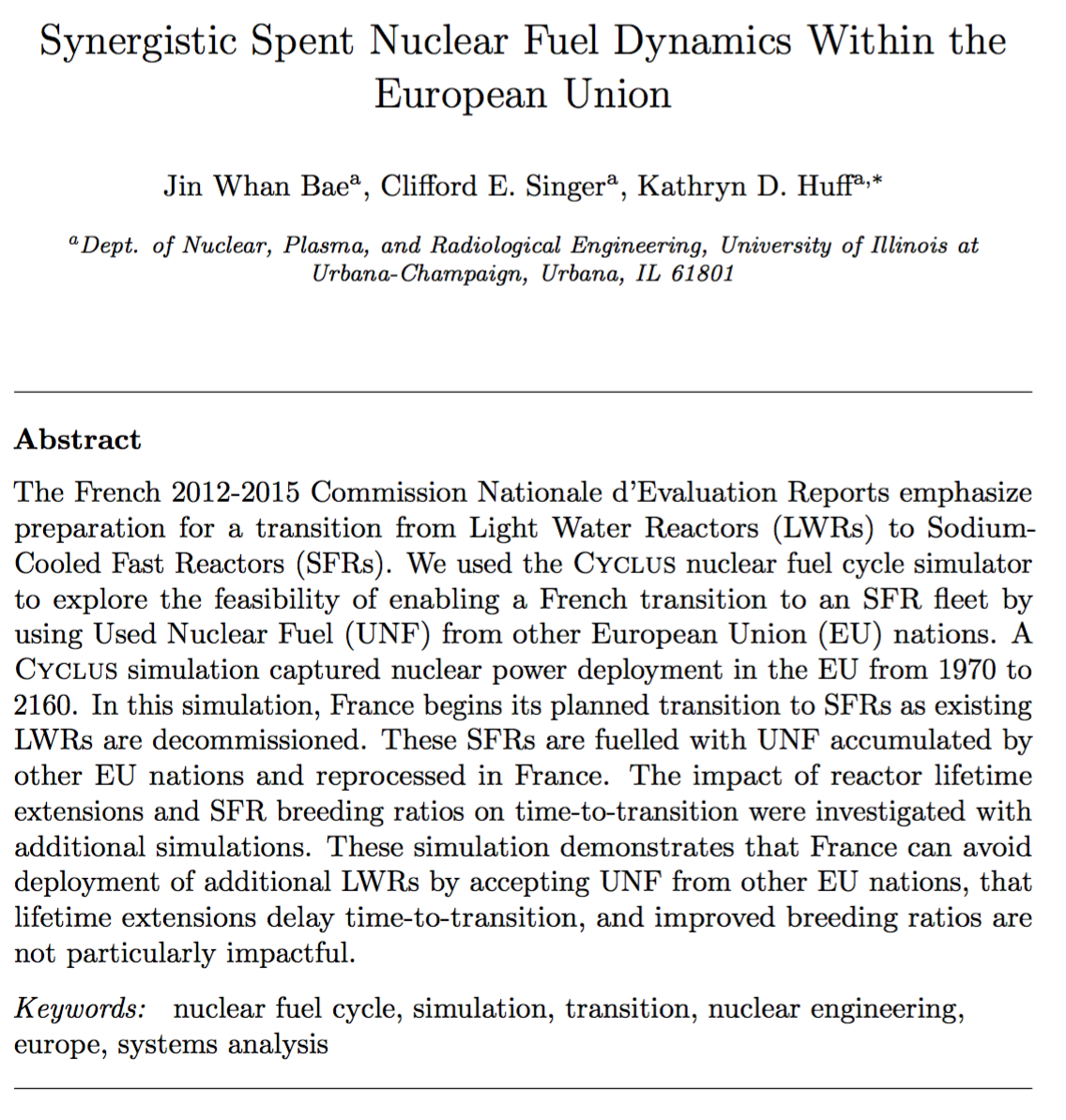### Transition Analysis

Can France transition to SFRs faster by reprocessing spent fuel from other EU nations?

• French LWR to SFR Transition
• Other EU Nations send all SNF to France
• $T_0 = 1970$
• $T_f <= 2150$
• Annual nuclear energy demand growth: 0%
• Sensitivity Analysis: Legacy LWR lifetimes vs. time to transition.
• Sensitivity Analysis: SFR breeding ratio vs. time to transition.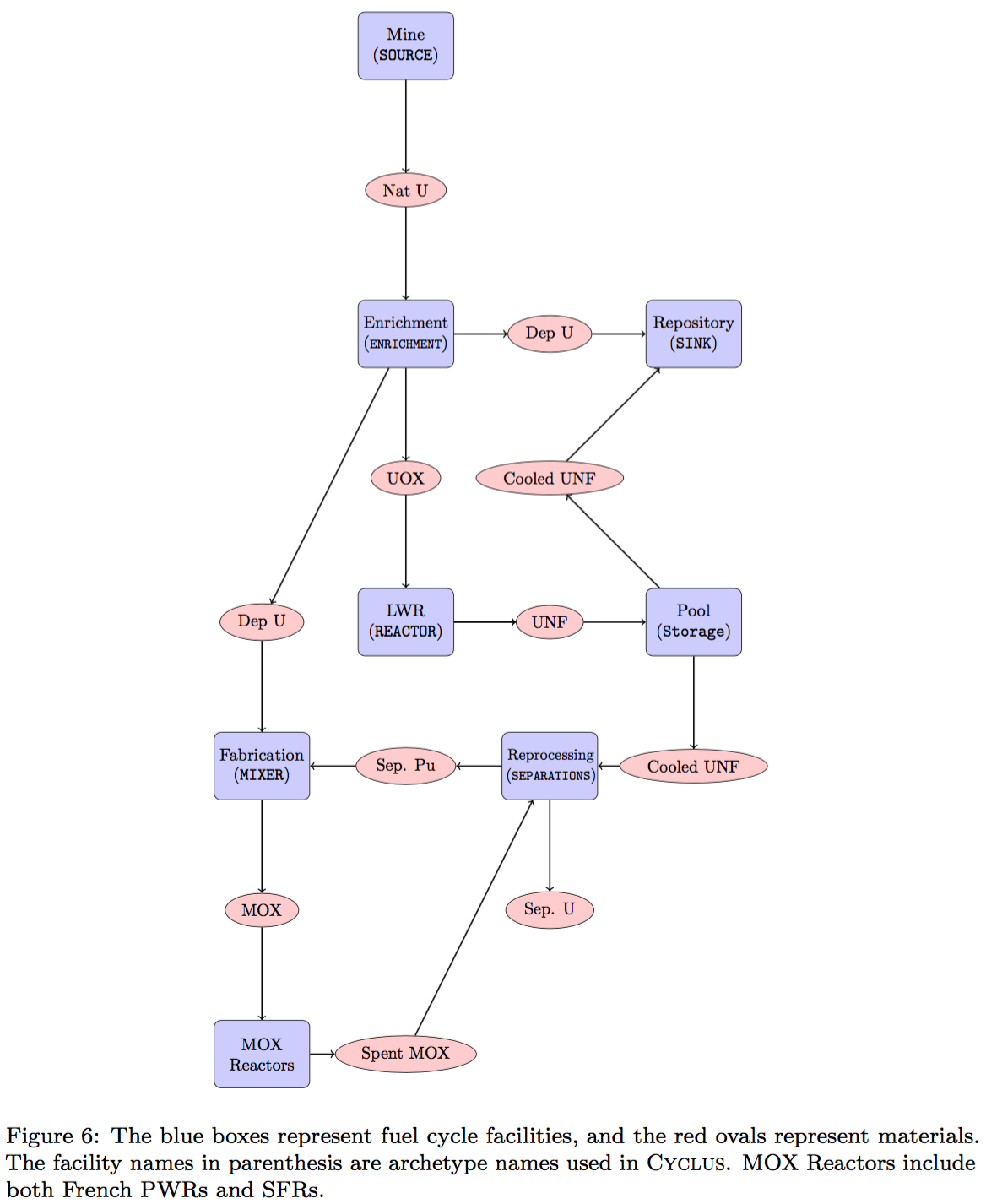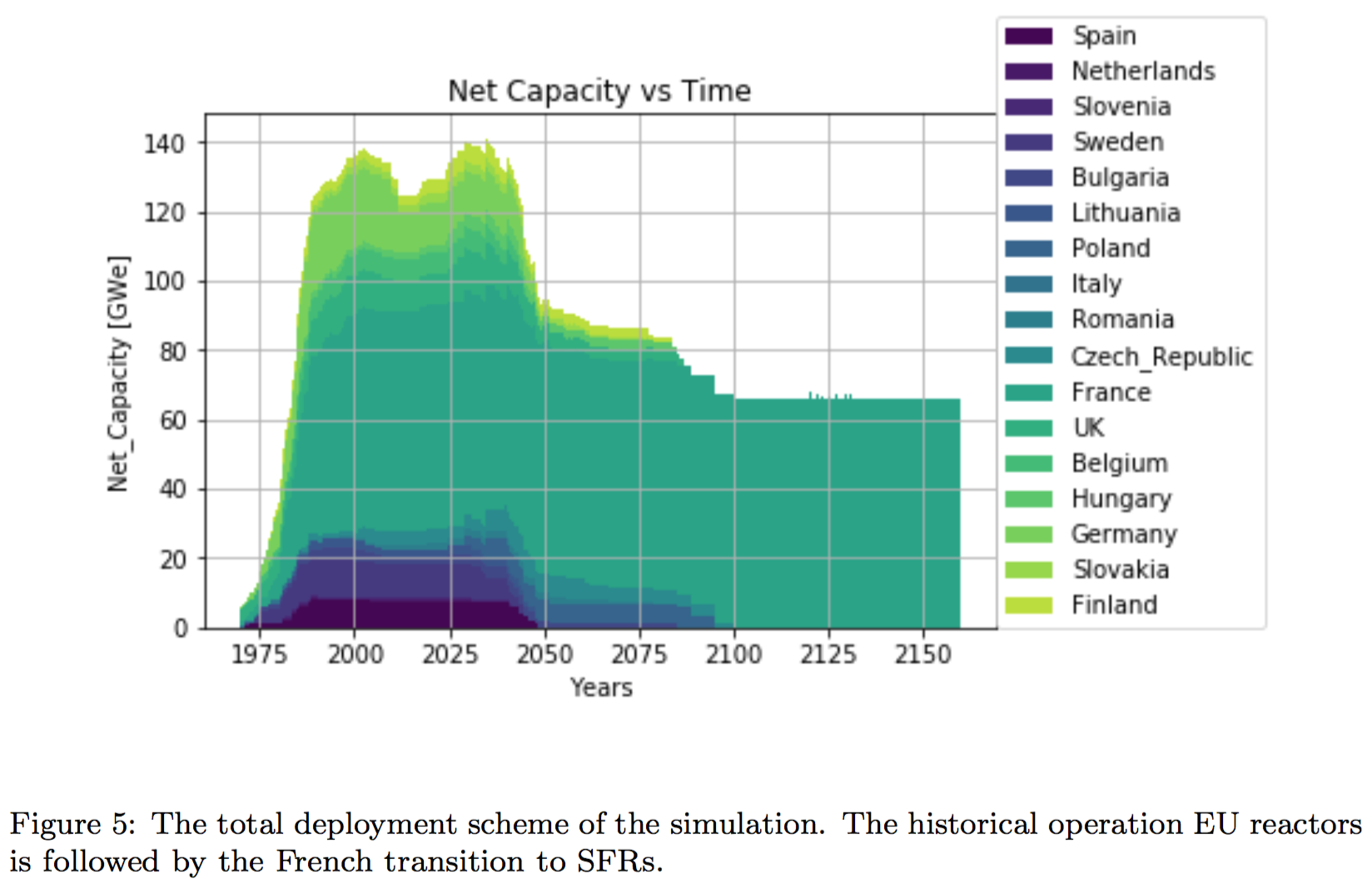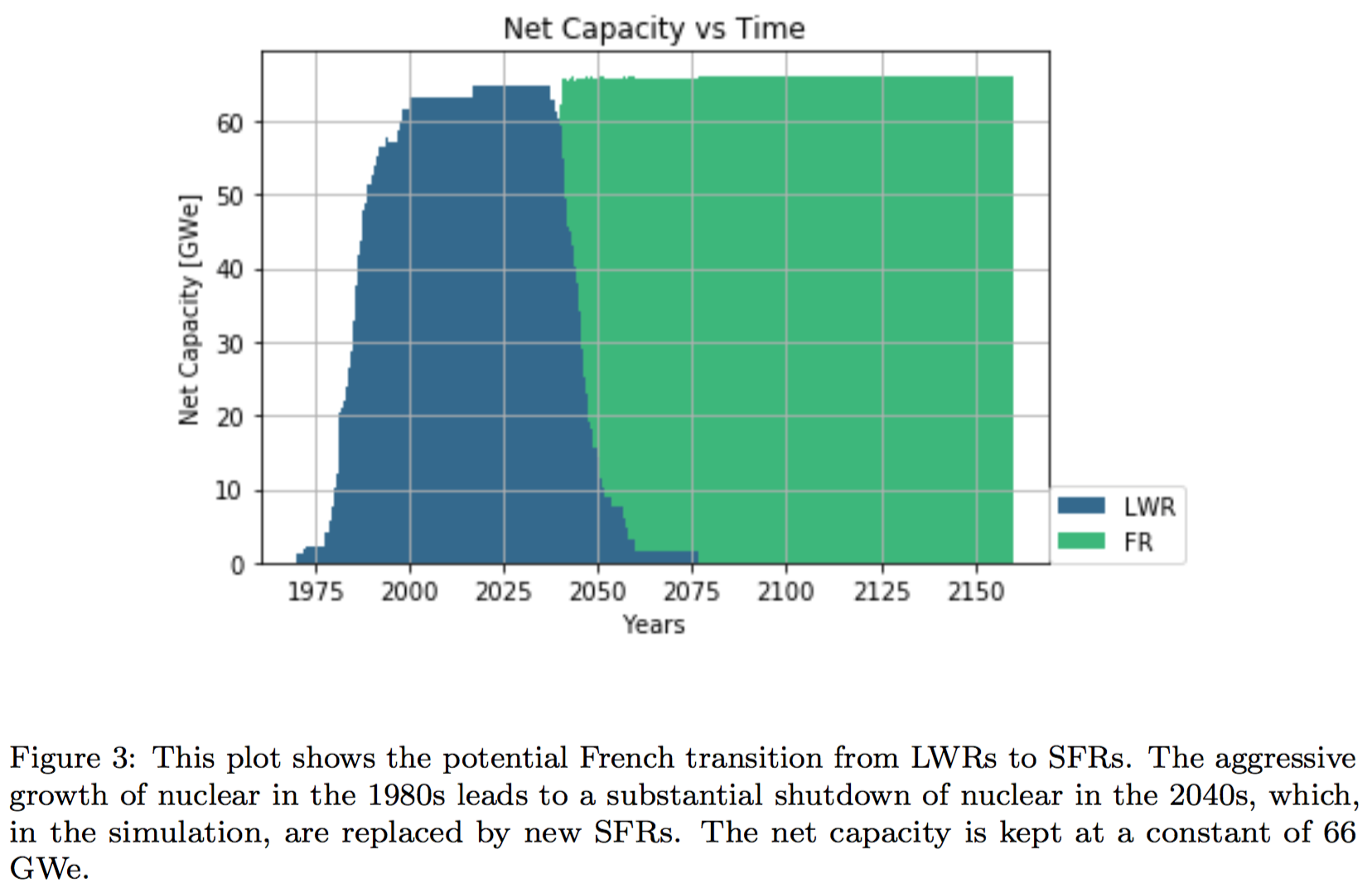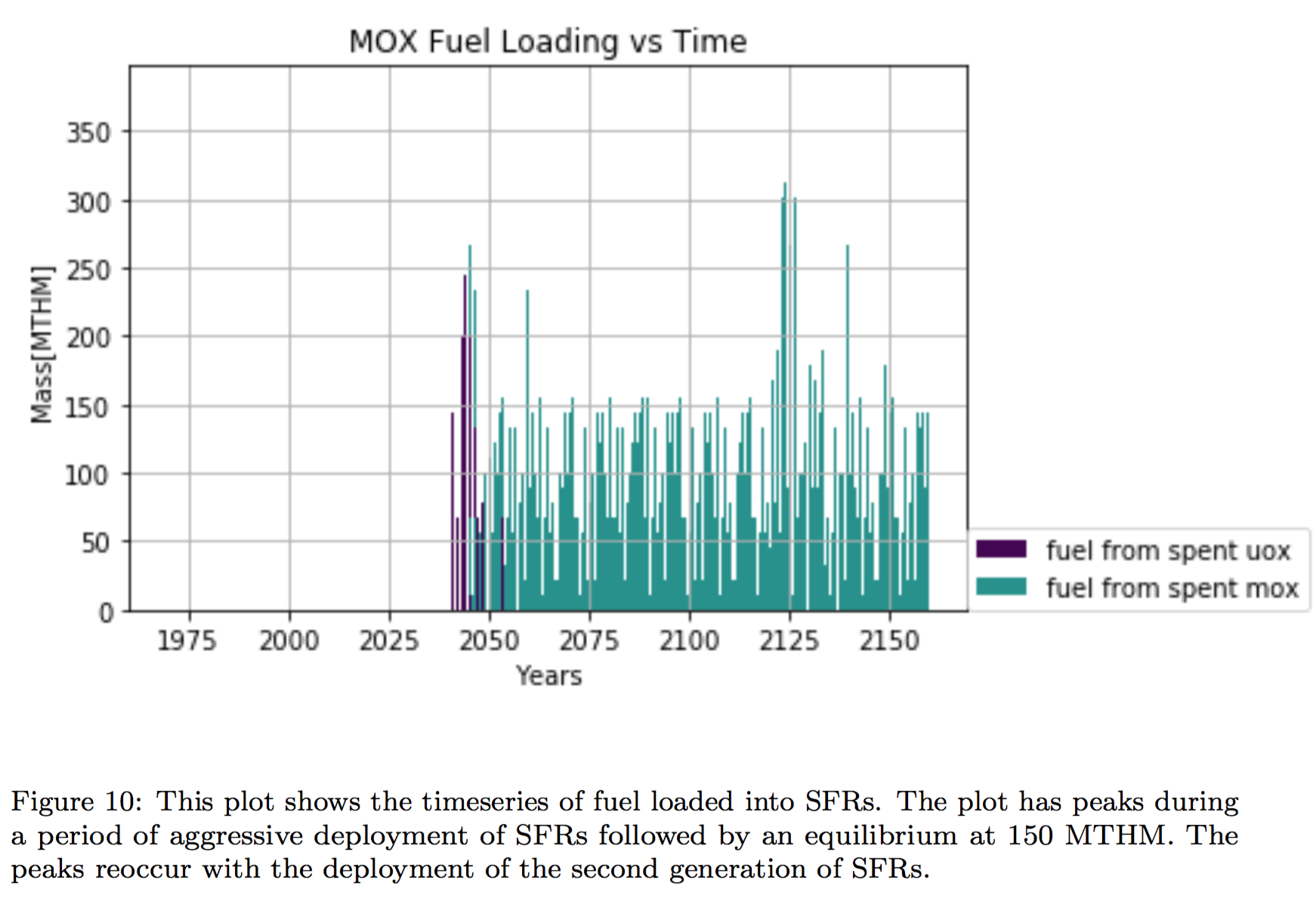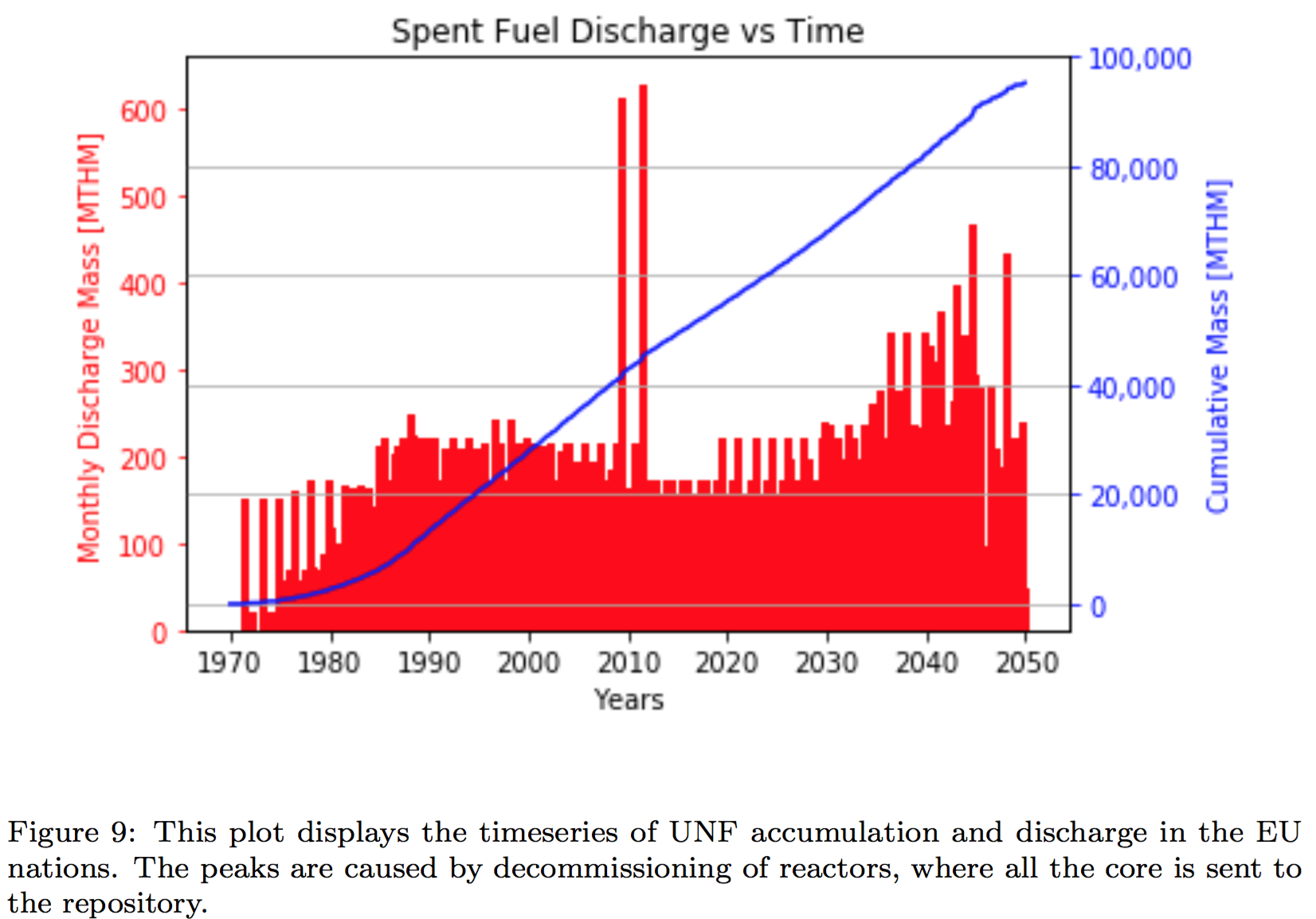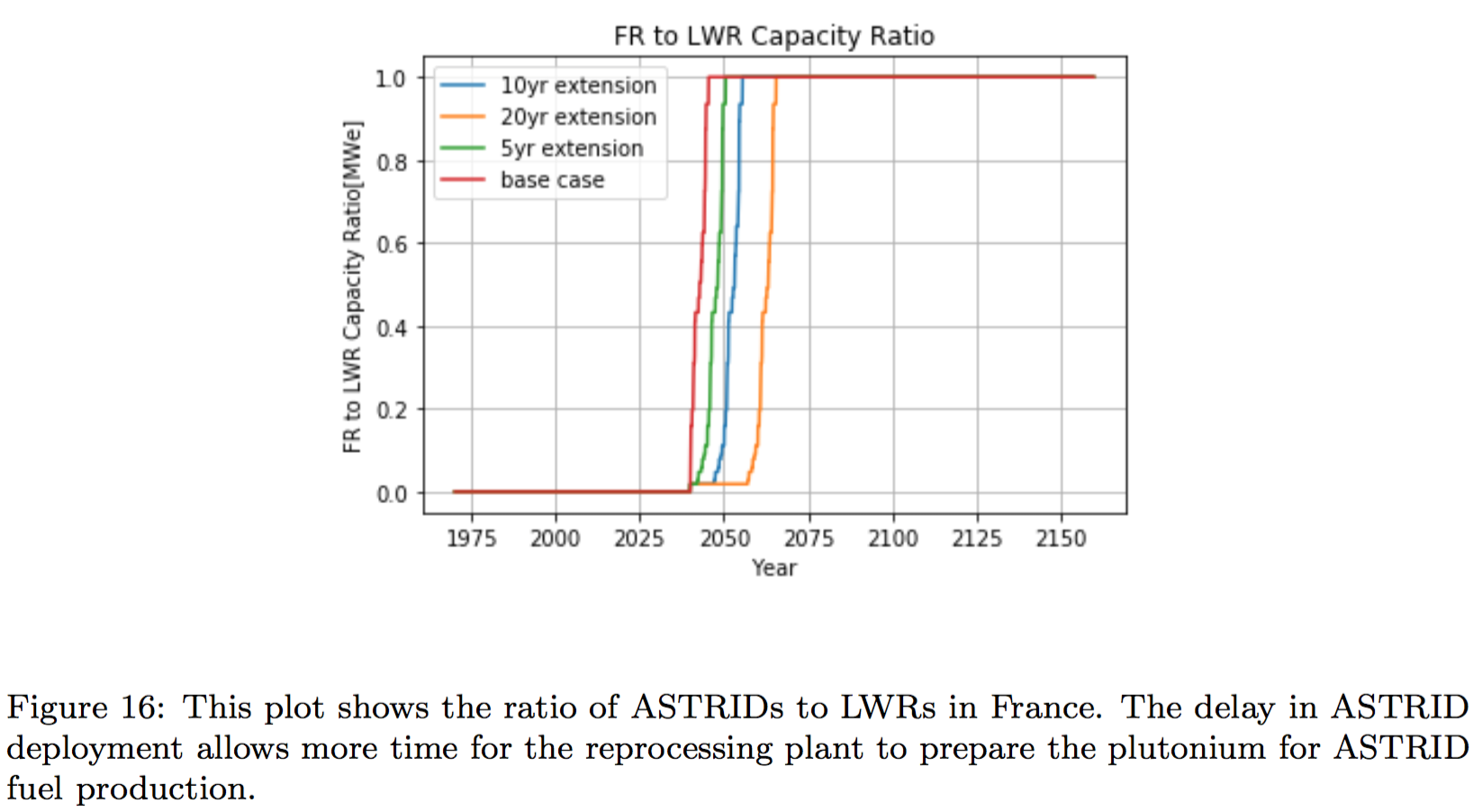### Conclusions

• The collaborative strategy speeds up the french transition.
• In particular, the nations planning aggressive nuclear reduction will be able phase out nuclear without constructing a permanent repository.
• Lifetime extensions slow down the transition, especially long extensions.
• Increasing the lifetime of French LWRs decreases reprocessing demand

### A Few of My Favorite Things

• C++, Python, Fortran
• Serpent, MOOSE, ORIGEN
• xml, markdown, rst, $\LaTeX$
• Doxygen, sphinx
• CMake, conda, macports